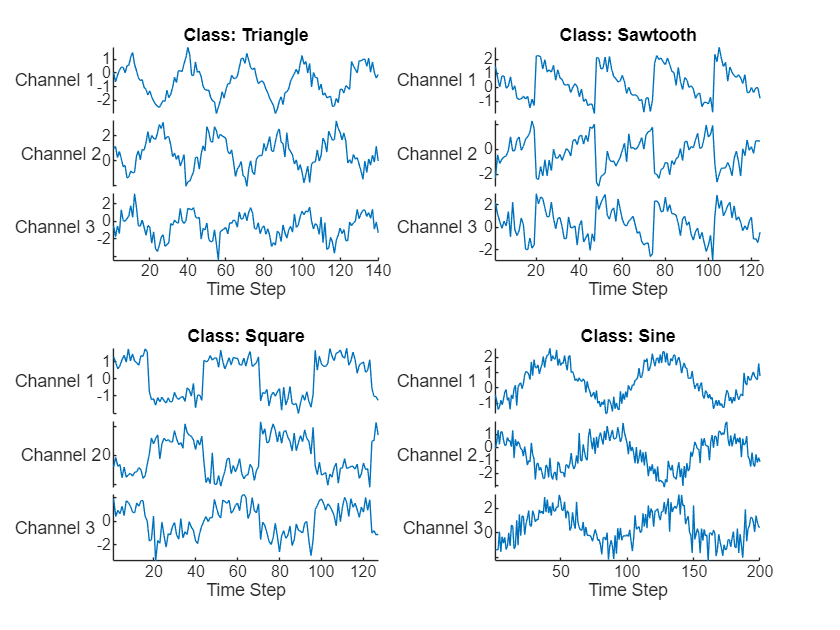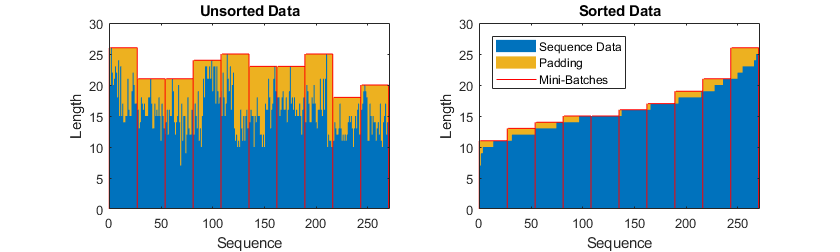# Sequence Classification Using Deep Learning

This example shows how to classify sequence data using a long short-term memory (LSTM) network.

To train a deep neural network to classify sequence data, you can use an LSTM network. An LSTM network enables you to input sequence data into a network, and make predictions based on the individual time steps of the sequence data.

This example uses the Waveform data set. This example trains an LSTM network to recognize the type of waveform given time series data. The training data contains time series data for four types of waveform. Each sequence has three channels and varies in length.

Load the example data from `WaveformData`. The sequence data is a `numObservations`-by-1 cell array of sequences, where `numObservations` is the number of sequences. Each sequence is a `numChannels`-by`-numTimeSteps` numeric array, where `numChannels` is the number of channels of the sequence and `numTimeSteps` is the number of time steps of the sequence. The label data is a `numObservations`-by-`1` categorical vector.

`load WaveformData `

Visualize some of the sequences in a plot.

```numChannels = size(data{1},1); idx = [3 4 5 12]; figure tiledlayout(2,2) for i = 1:4 nexttile stackedplot(data{idx(i)}',DisplayLabels="Channel "+string(1:numChannels)) xlabel("Time Step") title("Class: " + string(labels(idx(i)))) end```Set aside data for testing. Partition the data into a training set containing 90% of the data and a test set containing the remaining 10% of the data. To partition the data, use the `trainingPartitions` function, attached to this example as a supporting file. To access this file, open the example as a live script.

```numObservations = numel(data); [idxTrain,idxTest] = trainingPartitions(numObservations, [0.9 0.1]); XTrain = data(idxTrain); TTrain = labels(idxTrain); XTest = data(idxTest); TTest = labels(idxTest);```

During training, by default, the software splits the training data into mini-batches and pads the sequences so that they have the same length. Too much padding can have a negative impact on the network performance.

To prevent the training process from adding too much padding, you can sort the training data by sequence length, and choose a mini-batch size so that sequences in a mini-batch have a similar length. The following figure shows the effect of padding sequences before and after sorting data.Get the sequence lengths for each observation.

```numObservations = numel(XTrain); for i=1:numObservations sequence = XTrain{i}; sequenceLengths(i) = size(sequence,2); end```

Sort the data by sequence length.

```[sequenceLengths,idx] = sort(sequenceLengths); XTrain = XTrain(idx); TTrain = TTrain(idx);```

View the sorted sequence lengths in a bar chart.

```figure bar(sequenceLengths) xlabel("Sequence") ylabel("Length") title("Sorted Data")```### Define LSTM Network Architecture

Define the LSTM network architecture. Specify the input size to be the number of channels of the input data. Specify a bidirectional LSTM layer with 120 hidden units, and output the last element of the sequence. Finally, include a fully connected layer with an output size that matches the number of classes, followed by a softmax layer and a classification layer.

If you have access to full sequences at prediction time, then you can use a bidirectional LSTM layer in your network. A bidirectional LSTM layer learns from the full sequence at each time step. If you do not have access to the full sequence at prediction time, for example, if you are forecasting values or predicting one time step at a time, then use an LSTM layer instead.

```numHiddenUnits = 120; numClasses = 4; layers = [ ... sequenceInputLayer(numChannels) bilstmLayer(numHiddenUnits,OutputMode="last") fullyConnectedLayer(numClasses) softmaxLayer classificationLayer]```
```layers = 5×1 Layer array with layers: 1 '' Sequence Input Sequence input with 3 dimensions 2 '' BiLSTM BiLSTM with 120 hidden units 3 '' Fully Connected 4 fully connected layer 4 '' Softmax softmax 5 '' Classification Output crossentropyex ```

Specify the training options. Train using the Adam solver with a learn rate of 0.002 and a gradient threshold of 1. Set the maximum number of epochs to 150 and disable shuffling. The software, by default, trains on a GPU if one is available. Using a GPU requires Parallel Computing Toolbox and a supported GPU device. For information on supported devices, see GPU Computing Requirements (Parallel Computing Toolbox).

```options = trainingOptions("adam", ... InitialLearnRate=0.002,... MaxEpochs=150, ... Shuffle="never", ... GradientThreshold=1, ... Verbose=false, ... Plots="training-progress");```

### Train LSTM Network

Train the LSTM network with the specified training options by using `trainNetwork`.

`net = trainNetwork(XTrain,TTrain,layers,options);`### Test LSTM Network

Classify the test data and calculate the classification accuracy of the predictions.

`XTest(1:3)`
```ans=3×1 cell array {3×127 double} {3×180 double} {3×193 double} ```

The LSTM network `net` was trained using mini-batches of sequences of similar length. Ensure that the test data is organized in the same way. Sort the test data by sequence length.

```numObservationsTest = numel(XTest); for i=1:numObservationsTest sequence = XTest{i}; sequenceLengthsTest(i) = size(sequence,2); end [sequenceLengthsTest,idx] = sort(sequenceLengthsTest); XTest = XTest(idx); TTest = TTest(idx);```

Classify the test data and calculate the classification accuracy of the predictions.

```YTest = classify(net,XTest); acc = mean(YTest == TTest)```
```acc = 0.8400 ```

Display the classification results in a confusion chart.

```figure confusionchart(TTest,YTest)```